# 环境

window8.1，python3.7，Tableau2019.1

# 正文

## 1. 项目背景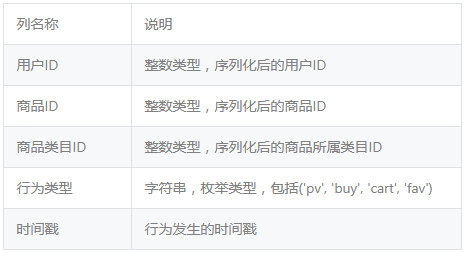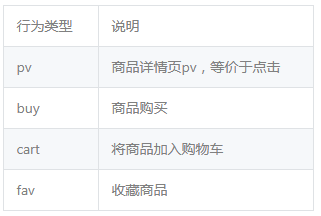## 2. 明确分析思路和目的

--分析思路：从what、why和how这三个角度对用户行为进行分析，即什么是用户行为分析？为什么进行用户行为分析？如何进行用户行为分析？而其中的why作为我们的重点解释对象。
--分析目的：通过对淘宝用户的点击、加购、收藏、购买行为进行深入研究，让企业或者店铺更好地了解用户行为习惯，为网络营销提供指导意义，也为取得新成绩打下坚实的基础。

### 2.3 how 如何进行用户行为分析?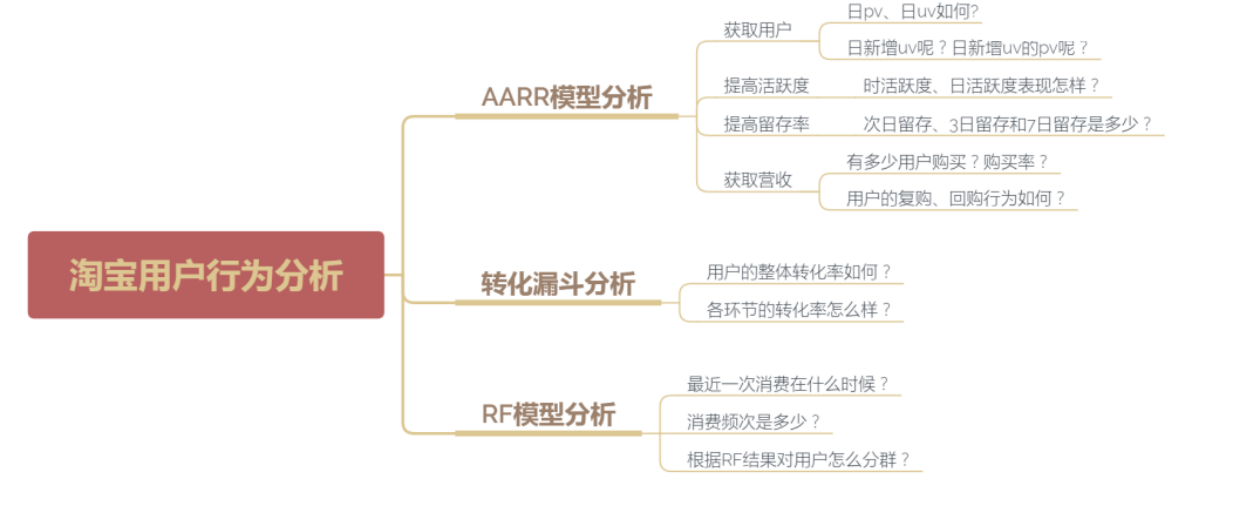## 3. 数据概览

### 3.1 读取数据

import pandas as pd
names=['用户ID','商品ID','商品类目ID','行为类型','时间戳'])

### 3.2 查看数据信息

df.info()

<class 'pandas.core.frame.DataFrame'>
RangeIndex: 5000000 entries, 0 to 4999999
Data columns (total 5 columns):

dtypes: int64(4), object(1)
memory usage: 190.7+ MB

### 3.3 描述性统计

df.describe()
df.describe(include=['O'])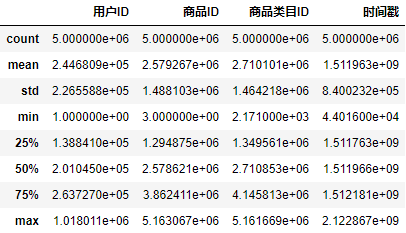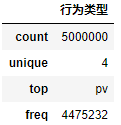## 4. 数据预处理

### 4.1 重复值处理

df.drop_duplicates(keep='last',inplace=True)

### 4.2 缺失值处理

df.isnull().sum()

dtype: int64

### 4.3 异常值处理

import time
start_time = time.mktime(time.strptime("2017-11-25 00:00:00",'%Y-%m-%d %H:%M:%S'))
end_time = time.mktime(time.strptime("2017-12-03 23:59:59",'%Y-%m-%d %H:%M:%S'))
df = df[(df.时间戳 >=int(start_time)) & (df.时间戳<= int(end_time))]

### 4.4 数据清洗

df['时间戳'] = df.时间戳.apply(lambda x: datetime.datetime.fromtimestamp(x))

df['日期'] = df.时间戳.dt.date
df['时间'] = df.时间戳.dt.time
df['小时'] = df.时间戳.dt.hour

df.head()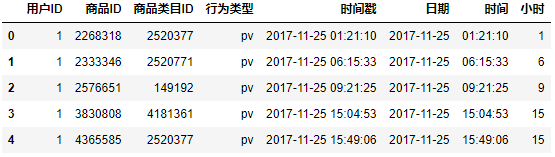## 5. AARR模型分析

### 5.1 获取用户

1.日pv、日人均pv和日uv

import numpy as np
from pyecharts import Line, Overlap
pv_day = df[df.行为类型 == 'pv'].groupby('日期')['行为类型'].count()
uv_day = df[df.行为类型 == 'pv'].drop_duplicates(['用户ID','日期']).groupby('日期')['用户ID'].count()
attr = pv_day.index
v1 = pv_day.values
v2 = uv_day.values
line1 = Line('日pv、日人均pv和日uv对比图')
mark_line=['average'], legend_pos='right')
mark_line=['average'], yaxis_max=100, yaxis_name='pv', yaxis_name_pos='end',
yaxis_name_gap=15, legend_pos='right', legend_top='3%')
line2 = Line()
mark_line=['average'], yaxis_name='uv', yaxis_name_pos='end', yaxis_name_gap=15)
overlap = Overlap()
overlap.render()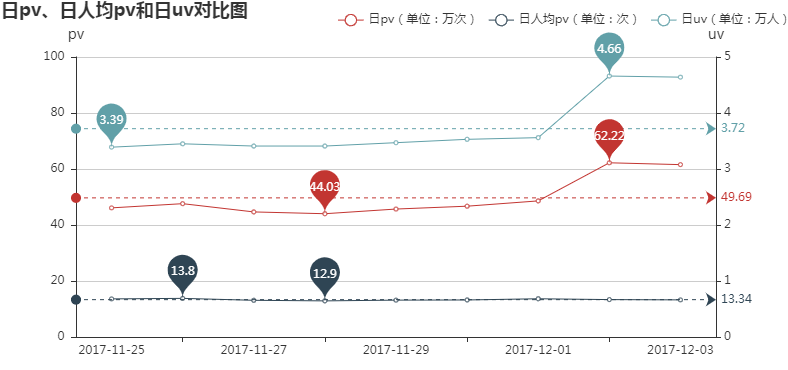--日pv和日uv两者走势相类似，也进一步说明日人均pv波动较平缓，其平均水平为13.34；
--日pv和日uv均呈现上升趋势，且均在12月2日突然升高至九日内最高水平，而12月2日是周六，但11月25日也是周六，因此可能不是周末的原因，又由于12月2日距离双十一较近且多数人会在双十一购买近期所需物品，因此初步推测12月2日~3日的突然升高是因为商家进行促销、宣传推广等活动。

2.日新增uv和日新增uv的pv

from pyecharts import Bar, Line, Overlap
from copy import deepcopy
import datetime
df_pv = df[df.行为类型 == 'pv']
s = set()
days = []
nums = []
for date in df_pv['日期'].unique():
num1 = len(s)
s1 = deepcopy(s)
ids = df_pv[df_pv.日期 == date]['用户ID'].values.tolist()
for i in ids:
num2  = len(s)
days.append(date)
nums.append(num2-num1)
df_new_uv = pd.DataFrame({'日期': days, '新增访客数': nums, '新增访客的浏览量': add_pv})

attr = df_new_uv.日期
v = df_new_uv.新增访客数
w = df_new_uv.新增访客的浏览量
bar = Bar('日新增uv和日新增uv的pv对比图')
bar.add('日新增uv', attr, v, is_label_show=True, yaxis_formatter=" 人", legend_pos='right', legend_top='3%', label_pos='outside')
line = Line()
line.add('日新增uv的pv', attr, w, yaxis_formatter=" 次",is_label_show=True, yaxis_max=700000, label_pos='inside')
overlap = Overlap()
overlap.render()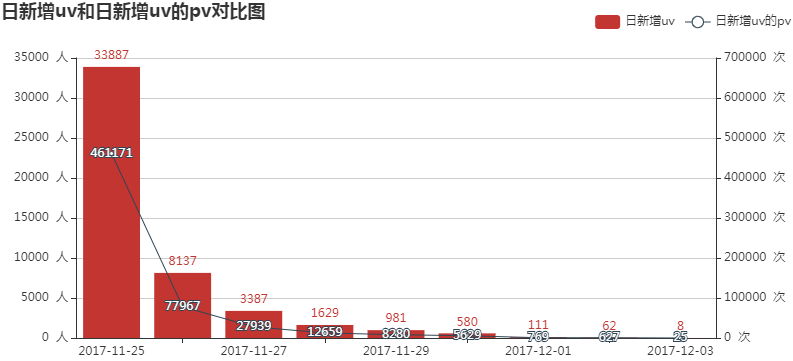### 5.2 提高活跃度

1.时活跃用户数

hour_active = df.drop_duplicates(['用户ID', '小时']).groupby('小时')['用户ID'].count()
line = Line('时活跃用户折线图')
mark_line=['average'], yaxis_formatter=' 人')
line.render()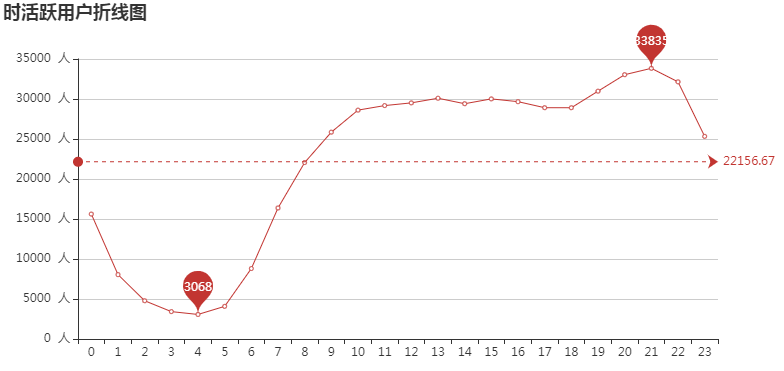19时~22时为用户活跃高峰期， 而2时~5时则为用户活跃低峰期，可在用户活跃高峰期加大活动宣传力度。

2.日活跃用户数

day_active = df.drop_duplicates(['用户ID', '日期']).groupby('日期')['用户ID'].count()
day_active
line = Line('日活跃用户折线图')
line.add('', day_active.index, day_active.values, is_label_show=True, yaxis_formatter=' 人')
line.render()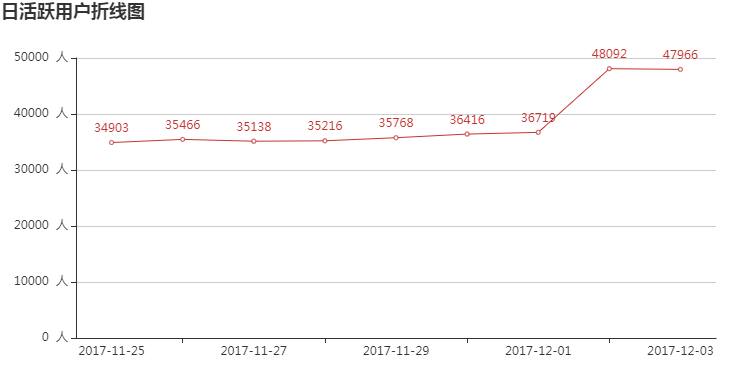### 5.3 提高留存率

from datetime import timedelta
def get_nday_retention_rate(df, n):
users2 = set()
days = []
nday_retentions = []
dates = df.日期.unique()[:-n]
for date in dates:
users1 = deepcopy(users2)
ids = df[df.日期 == date].用户ID.values.tolist()
for i in ids:
users = users2 - users1
nday_users = df[df.日期 == date + timedelta(days=n)].用户ID.unique()
counts = 0
for nday_user in nday_users:
if nday_user in users:
counts += 1
nday_retention_rate = counts / len(users)
nday_retentions.append(nday_retention_rate)
days.append(date)
df_retention_rate = pd.DataFrame({'日期': days, 'n日留存率': nday_retentions})
return df_retention_rate

retention_rate1 = get_nday_retention_rate(df, 1)
retention_rate2 = get_nday_retention_rate(df, 2)
retention_rate3 = get_nday_retention_rate(df, 3)
retention_rate4 = get_nday_retention_rate(df, 4)
retention_rate5 = get_nday_retention_rate(df, 5)
retention_rate6 = get_nday_retention_rate(df, 6)
retention_rate7 = get_nday_retention_rate(df, 7)
line = Line('留存率对比分析图')
line.render()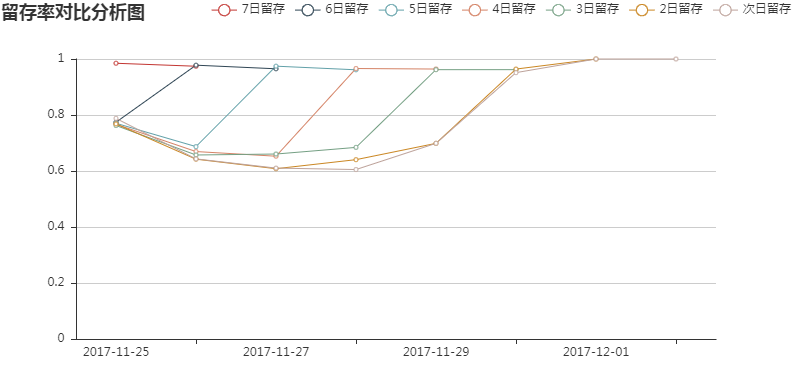--就时间窗口来说，次日留存和3日留存均表现出先减后增的趋势，而7日留存则相比之前略有减少；
--就某一天来说，11月25日新增的活跃用户3日留存<次日留存<7日留存，11月26日新增的活跃用户次日留存<3日留存<7日留存，且其他日期3日留存均大于次日留存。

### 5.4 获取营收

1.时购买行为

hour_buy_user_num = df[df.行为类型 == 'buy'].drop_duplicates(['用户ID', '小时']).groupby('小时')['用户ID'].count()
hour_active_user_num = df.drop_duplicates(['用户ID', '小时']).groupby('小时')['用户ID'].count()
line1 = Line('时购买行为分析')
line1.add('购买人数', attr, v1, mark_point=['max', 'min'], yaxis_formatter=' 人')
line2 = Line()
line2.add('购买率', attr, np.around(v2, 3), mark_point=['max', 'min'])
overlap = Overlap()
overlap.render()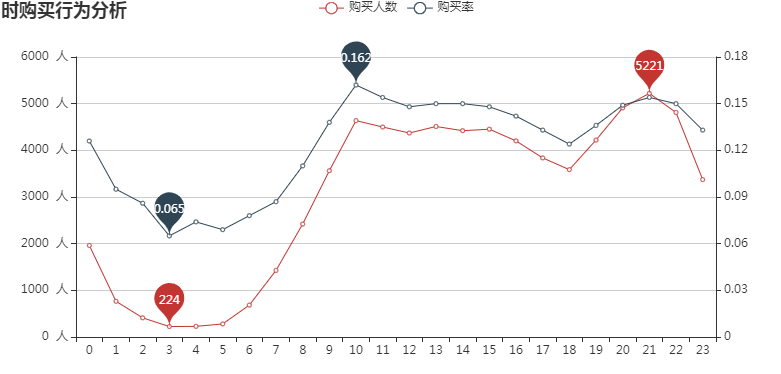2.日购买行为

day_buy_user_num = df[df.行为类型 == 'buy'].drop_duplicates(['用户ID', '日期']).groupby('日期')['用户ID'].count()
day_active_user_num = df.drop_duplicates(['用户ID', '日期']).groupby('日期')['用户ID'].count()
line1 = Line('日购买行为分析')
line1.add('购买人数', attr, v1, mark_point=['max', 'min'], yaxis_formatter=' 人')
line2 = Line()
line2.add('购买率', attr, np.around(v2, 3), mark_point=['max', 'min'])
overlap = Overlap()
overlap.render()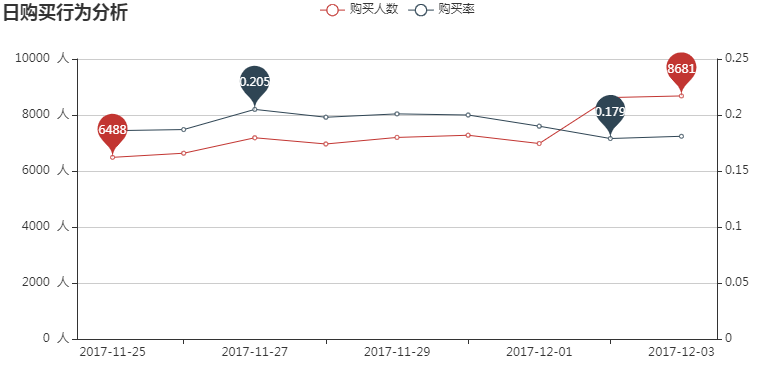3.九日复购率

df_rebuy = df[df.行为类型 == 'buy'].drop_duplicates(['用户ID', '时间戳']).groupby('用户ID')['时间戳'].count()
df_rebuy[df_rebuy >= 2].count() / df_rebuy.count()

0.6323078771856036

4.三日复购率和回购率

#计算复购率
for m, n in zip(range(1, 10), df.日期.unique()):
if m % 3 == 0:
df.loc[(df.日期 + timedelta(days=0) <= n) & (df.日期 + timedelta(days=2) >= n), '日期1'] = n
df.日期1.unique()
#计算回购率
days = []
for i in range(0, 2):
counts = 0
for user in users_id:
counts += 1
#绘制折线图
line = Line('用户每三日复购和回购行为分析')
line.render()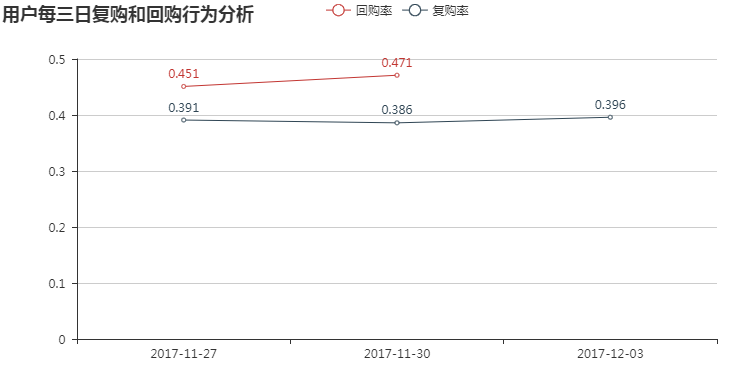--用户回购率整体高于复购率，其波动性也明显强于复购率；
--用户复购率呈现先减后增的趋势，而用户回购率则是增加趋势 , 即第二周期购买用户的忠诚度较第一期高，整体说明用户忠诚度在增加。

## 6. 转化漏斗模型分析

from pyecharts import Funnel
pv_users = df[df.行为类型 == 'pv']['用户ID'].count()
fav_users = df[df.行为类型 == 'fav']['用户ID'].count()
cart_users = df[df.行为类型 == 'cart']['用户ID'].count()
attr = ['点击', '加入购物车', '收藏', '购买']
values = [np.around((pv_users / pv_users * 100), 2),
np.around((cart_users / pv_users * 100), 2),
np.around((fav_users / pv_users * 100), 2),
np.around((buy_users / pv_users * 100), 2)]
funnel = Funnel("用户行为转化漏斗", title_pos='center')#width=600, height=400,
"",
attr,
values,
is_label_show=True,
label_formatter = '{b} {c}%',
label_pos="outside",
is_legend_show = False,
)
funnel.render()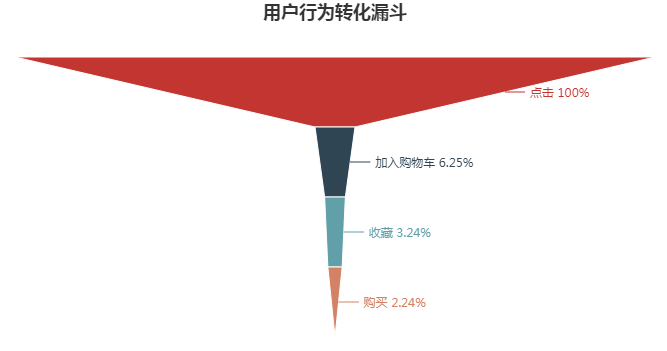--总的点击量中，有6.25%加入购物车，有3.24%收藏，而到最后只有2.24%购买，整体来看，购买的转化率最低，有很大的增长空间；
--就颜色来看，红色部分的变化最大，即“点击-加入购物车“这一环节的转化率最低，按照“点击-加入购物车-收藏-购买”这一用户行为路径，我们可通过优化“点击-加入购物车”这一环节进而提升购买的转化率。

## 7. RF模型分析

R：Recency（最近一次消费），F：Frequency（消费频次），M：Monetary（消费金额）

from datetime import date
nowdate = date(2017, 12, 5)
rf_module['recency_avg'] = rf_module.recency.mean()
rf_module['frequency_avg'] = rf_module.frequency.mean()
rf_module.to_excel(r'E:\Data\data2.xlsx')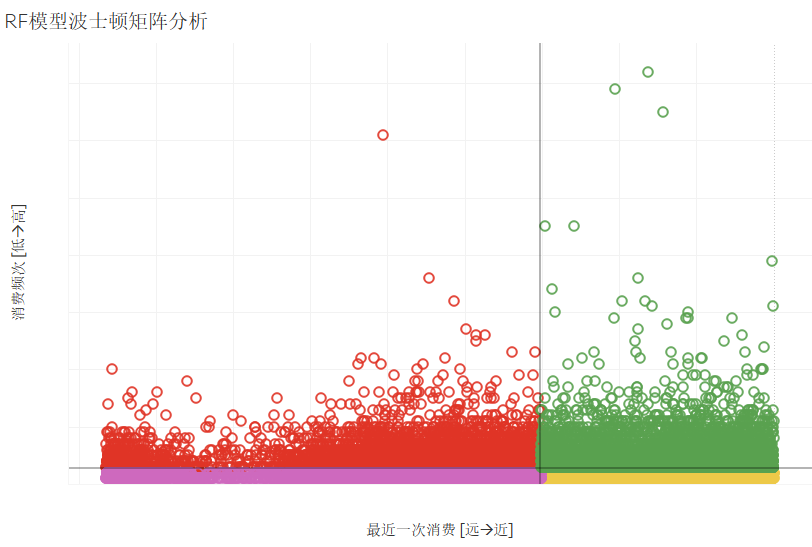--第一象限（重要价值用户）：该象限用户消费频次高、最近一次消费近，应当继续保持并给予支持；
--第二象限（重点保持用户）：该象限用户消费频次高、最近一次消费远，可通过电话、短信等方式主动联系促进最近消费；
--第三象限（重点挽留用户）：该象限用户消费频次低、最近一次消费远，可通过赠送礼品、加大折扣等方式进行挽回；
--第四象限（重点发展用户）：该象限用户消费频次低、最近一次消费近，可通过发放优惠券、捆绑销售等方式增加用户购买频次。

# 总结

1. 对比12月2日~12月3日与上一个周末的日pv、日uv和日活跃用户数，均发现有明显的上升趋势，且上升均是由老用户带来的，反映出老用户忠诚度表现不错，同时也说明了这两天的促活活动产生了效果；

2. 在每天的19时~22时为用户活跃高峰期，购买人数也最多，购买率略低；而在10时用户活跃度较低，购买人数也略低，但用户购买率却最高，因此就购买率来说，10时活跃用户产生的价值较高，应当对10时活跃用户的活动力度继续保持并给予支持，重点发展19~22时的活跃用户。

3. 在“点击-加入购物车-收藏-购买”转化漏斗中：就整体来说，购买转化率为2.24%表现最差，有很大的增长空间；就各环节来说，“点击-加入购物车”环节的转化率为6.25%表现最低，可以通过优化这一环节的转化率，来提升整体的购买转化率。

4. 商家可根据RF模型的分析结果，对不同群体的用户进行精准营销，达到利益最大化。©️2019 CSDN 皮肤主题: 编程工作室 设计师: CSDN官方博客